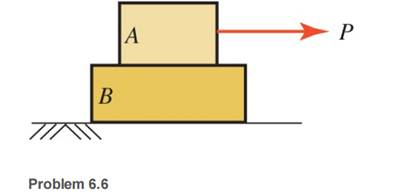### Create an Account

Already have account?

### Forgot Your Password ?

Home / Questions / Block A in the figure shown has a mass of 10 kg and block B has a mass of 20 kg The coeffi...

# Block A in the figure shown has a mass of 10 kg and block B has a mass of 20 kg The coefficients of static friction are 05 between the two blocks and 015 between the lower block and the supporting

Block A in the figure shown has a mass of 10 kg and block B has a mass of 20 kg. The coefficients of static friction are 0.5 between the two blocks and 0.15 between the lower block and the supporting surface. Calculate the force P that will cause motion to impend.Jun 26 2020 View more View Less

#### Answer (Solved)Subscribe To Get Solution